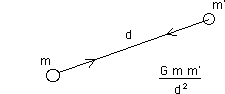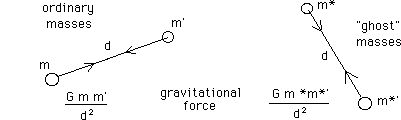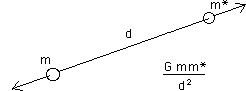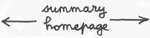1

Introduction.

Our purpose is to present some new insights on astrophysics, cosmology and elementary particles theory.

The underlying ideas are relatively simple. They can be expressed into three different forms :

- In a specialist's language.

- Schematically.

- In an ordinary way.

One can write ordinary texts on science following different methods.

...In general the writer of ordinary books, devoted to science, offers pretty images, in order to stimulate the reader's imagination. But, by the end of the book, he has not gained very much.

Using images, I mean, geometry, which greatly invaded physics since one century ago, one can offer better food for brains.

...An adequate analogical conversion makes possible to evoke the main goals and paths of a theory. By the way, the reader gainS, at the end, solid knowledge in 2d geometry.

Newton and anti-Newton.

...Cosmic structures are organised by a fundamental force : gravitation. If the objects of the cosmos did not mutually attract, nothing would happen over there. The galaxies would not have formed, neither stars, or planets. We wouldn't be here to talk about it all. For an example, if the gravitational force was a repulsive force, it would keep the objects far away one from another. The cosmos would stay amorphous, flabby, which would be deadly boring.

The attraction's law is the Newton's law :
(1)G is the constant of gravitation. m and m' are two masses and d is the distance between them.

...As will be shown further, our model involves "normal masses m " and something we call "ghost mass m ". Galaxies, stars, planets, human beings, cats, are made of normal mass. Nobody knows if ghost cats exist.

...The main point is that matter and ghost matter interact only through the gravitational force. Out of this, they ignore each other. For example they cannot exchange photons, so that the ghost objects are invisible from our fold of the Universe. Conversely if a "ghost observer" exists, he cannot see us, nor our galaxies, stars, etc...

The interaction scheme is the following :
(2)Two ordinary masses attract each other, following the classical Newton's law.

Same thing for two ghost masses.

But an ordinary mass and a ghost mass repel each other, according to some sort of "Anti-Newton's" law :
(3)...We could pu two sets of mass points, corresponding to ordinary and ghost masses, in a computer, let them interact, and see what happens. But the physicist will immediately argue :

- Your ghost matter has a negative mass m* < 0 !

In effect, if we reverse the sign of one mass in the Newton's law :
(5)the sign of the force changes....But if we change the sign of the two masses, the force is unchanged. Then one would tend to conclude that in this model we have simply two kinds of masses : one positive and the other negative, for it takes into account the suggested force's schema.

...But negative masses m* < 0 goes with m*c2 < 0 energies, which was investigated first by the french mathematician Jean-Marie Souriau in his book :

Structure of Dynamic Systems ( first edition in France, 1973, pages 197 to 200 ) :
Birkhauser Ed. 1997.

...Negative energy objects arise a serious problem. In effect, what happens when they collide with positive energy ( positive mass ) object ?

...This does not correspond to matter anti-matter annihilation. As will be shown in Geometrical Physics B, 4 , anti-matter located in our universe owns a positive mass and positive energy. Their meeting gives photons. The two produced photons carry the total amount of energy of the matter and anti-matter particles, before their collision. If two objects with opposite energies could meet, collide, the result should be totally different. That would give .... nothing.

...In the very begining of the universe, according to the Standard Model, matter and anti-matter collide frequently, producing high energy photons. But, conversely, these high energy photons give pairs of matter anti-matter particles. The two processes balance each other, in the early universe. But if the Universe would have contained positive and negative masses, positive energy particles and negative energy particles, the two sub-sets would have simply cancelled each other. If the initial proportion would have been fifty-fifty, the whole universe would have disappeared.

That's for the physicists are so skeptical about negative mass particles.

...That's why there is a need for a schematic presentation of the model. As will be show further "it works as if the ghost matter own a negative mass". To approach this apparent paradox we have to learn some geometry.